It would be difficult to imagine today’s industrial growth without bearings. Every kind of machine that produces motion uses a bearing device to transmit motion and reduce friction. Bearings come in different types, but the ball bearing is among the most commonly used in several industries.

A ball bearing is a rolling-element bearing that uses balls to support a rotating shaft, permitting its movement with minimum wear and friction.

However, there is more to ball bearings than meets the eye. For instance, ball bearings have a unique working principle and come in different types. In addition, there are several fundamental calculations that must be understood before choosing a ball bearing for an application.

## The construction and working principle of ball bearings

Ball bearings are usually made as an assembly of four parts:

• A large outer ring (or stationary outer raceway)
• A smaller inner ring (or rotating inner raceway) attached to the rotating assembly or shaft
• Rows of balls trapped between the raceways of the two rings
• ·A cage or separator that retains the balls and prevents them from touching one another.

The construction of ball bearings capitalizes on the concept that rolling elements (like rows of balls) produce less friction than sliding elements. Therefore, when the inner ring rotates, it causes the balls to roll around the inner raceway while the outer ring remains stationary. This allows rotational movement with a very low coefficient of friction, making it ideal for applications that require high-speed movement of shafts with minimum wear and friction.

When ball bearings operate, forces (or loads) are imposed on the bearings by the equipment being driven by the shaft. These forces are typically separated into two components: axial load and thrust load. The axial load acts 90° to the shaft, while the thrust load acts along the centerline of the shaft. The design of ball bearings allows them to withstand these loads.

However, ball bearings have a relatively low load capacity, making them ideal for applications with smaller load requirements to prevent failure.

## Types of ball bearings

### 1. Deep groove bearings

Deep-groove bearings are designed to have a unique raceway, allowing them to support high loads and withstand high speeds. They are typically available in single-row and double-row designs, depending on the number of rows of balls in the bearing.

### 2. Axial bearings

Axial bearings are designed to handle purely axial loads. These ball bearings feature multiple raceways side by side.

### 4. Angular contact bearings

Angular contact bearings feature axially asymmetrical raceways to withstand combined loads (axial and radial loads). As a result, they are ideal in gearboxes, electric motors and pump applications.

## Important parameters and calculations for ball bearing selection

The selection of ball bearings for a particular application will depend on the load, size of the shaft, and housing space limitations, among other things. However, the basic bearing calculations required for the selection are detailed below.

### 1. Bearing rating life

Bearing rating life is the number of revolutions that 90% of a group of identical bearings will complete (or exceed) before fatigue is noted. The rating life of a ball bearing (in millions of revolutions) is calculated according to the equation: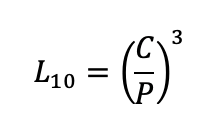Equation 1: Rating life of ball bearing, millions of revolutions.

Where:

L10 = Rating life of the ball bearing

C = the basic dynamic load rating (kN)

P = the equivalent dynamic bearing load (kN)

If the shaft speed and the bearing’s expected design life are known, then the bearing life rating (with no more than 10% failures) can be calculated with the equation below.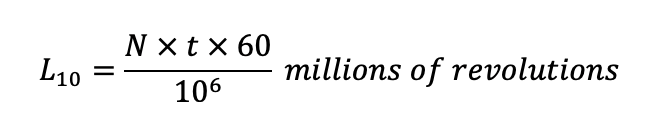Equation 2: Bearing life rating, millions of revolutions.

Where:

N = speed of the shaft (in revolutions per minute)

t = expected design life (in hours)

### 2. The static load capacity of bearing

The static load capacity (C0) of a bearing is the maximum permissible load that can be applied on a non-rotating bearing without impairing the bearing operation. It is the load that the bearing can endure before the bearing raceway causes permanent deformation of 0.01% of the ball diameter.

The basic static load can be calculated according to the following equations: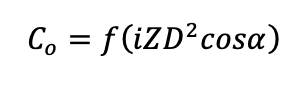Equation 3: Static load capacity of a ball bearing.

Where:

i = number of rows of balls in any one bearing

Z = number of balls per roll

D = ball diameter

= the nominal angle of contact

f = constant. For self-aligning ball bearing, f = 3.34 x 106. In contrast, f = 12.26x 106 can be used for radial and angular groove ball bearings.

Bearings will fail much earlier than their expected life rating if the static load is not considered during their selection. For instance, high shock loads that exceed the bearing’s static load capacity will likely cause bearing deformation and compromise its performance.

### 3. Equivalent static bearing load

Ball bearings are capable of withstanding a combination of radial load (Fr) and thrust/axial load (Fa). The equivalent static load (Po) is simply a theoretical load that causes the same deformation as the two loads when applied. It can be obtained using: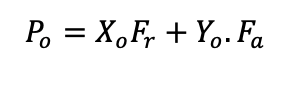Equation 4: Equivalent static bearing load.

Where:

Yo = static thrust load factor

The values can be obtained in the bearing specification table, typically provided by ball bearing manufacturers.

When ball bearings are subjected to axial and radial load, the equivalent dynamic load (Pe) is calculated using: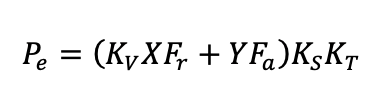Equation 5: Equivalent dynamic bearing load.

Where:

Kv = rotational factor

Ks = service factor

KT = temperature factor

These values are typically provided in standard tables and catalogs by manufacturers.

### 5. Bearing dynamic capacity

The bearing dynamic load capacity (C) is defined as the bearing load that will give a bearing life rating of one million revolutions. It is useful for calculating the basic life rating of bearings that rotate under load.

The basic dynamic load rating for ball bearings can be obtained using: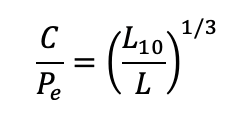Equation 6: Bearing dynamic capacity.

Where:

L10 = Rating life of the ball bearing (in millions of revolutions)

L = 1 million revolutions

C = the basic dynamic load rating